# 22. For the unity feedback system given in Figure P9.1 with G(S) = 5(+ 5)(s +...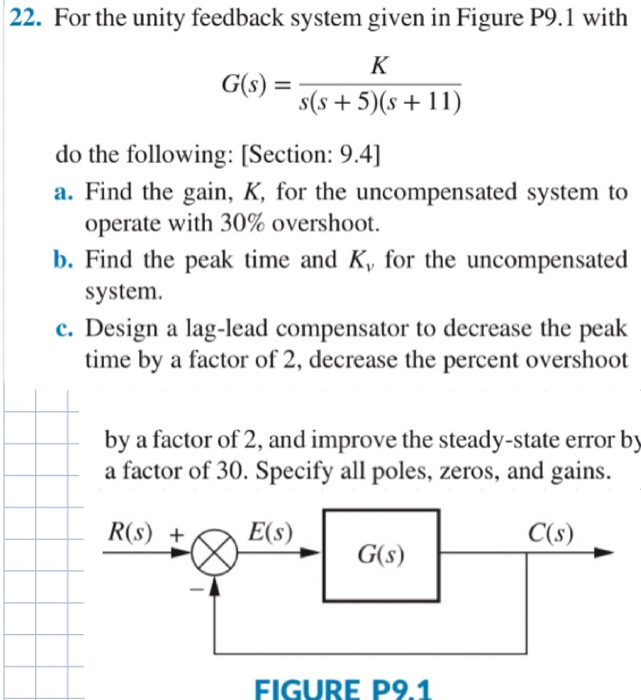22. For the unity feedback system given in Figure P9.1 with G(S) = 5(+ 5)(s + 11) do the following: [Section: 9.4] a. Find the gain, K, for the uncompensated system to operate with 30% overshoot. b. Find the peak time and K, for the uncompensated system, c. Design a lag-lead compensator to decrease the peak time by a factor of 2, decrease the percent overshoot by a factor of 2, and improve the steady-state error by a factor of 30. Specify all poles, zeros, and gains. R(s) + E(s) C(S) G(s) FIGURE P9.1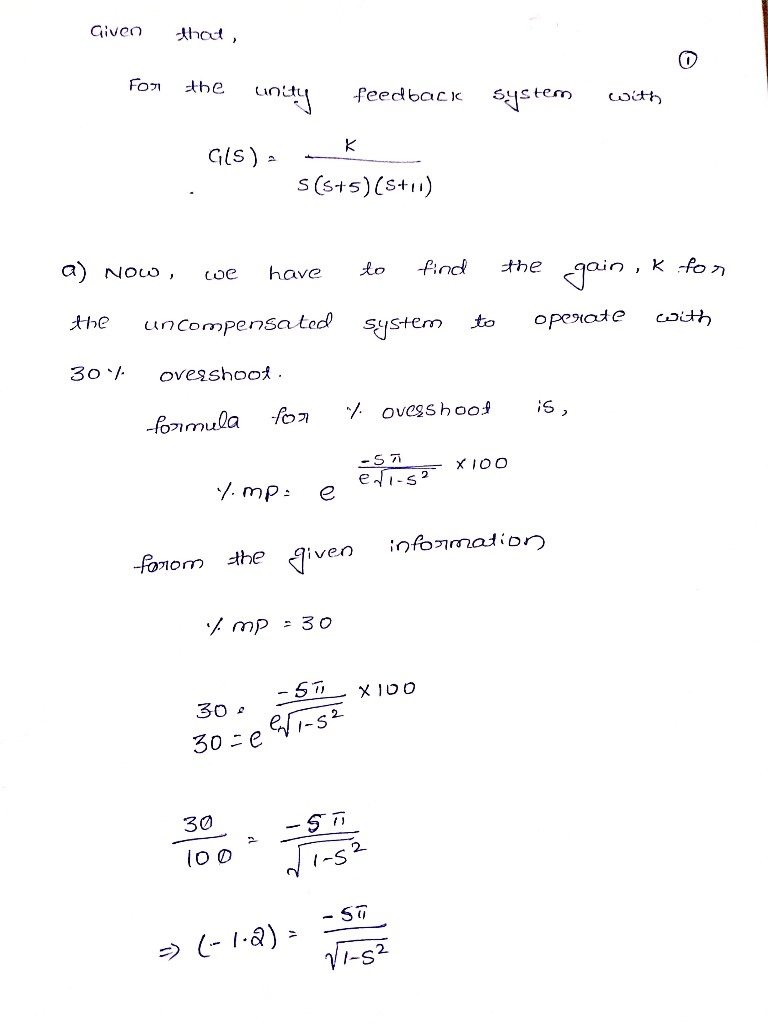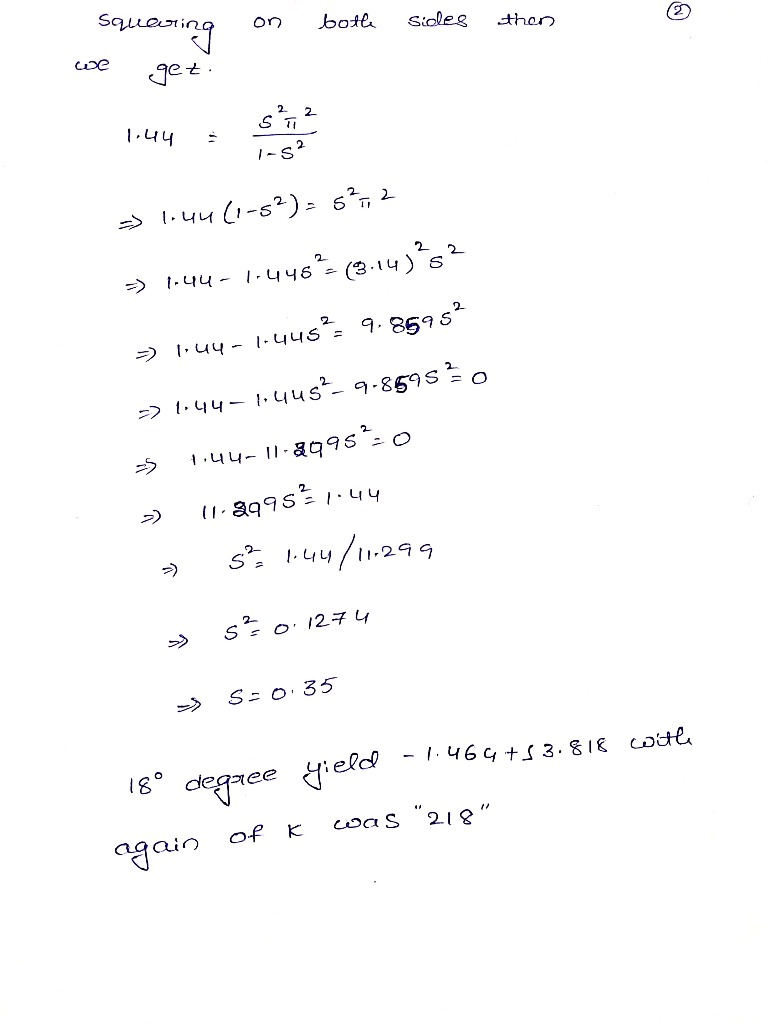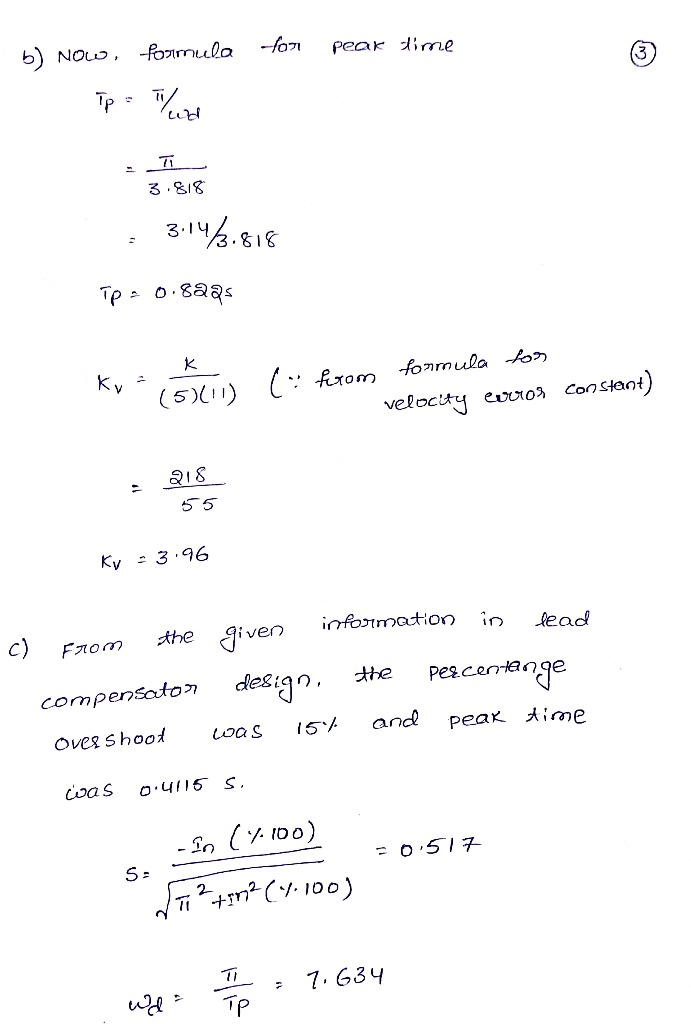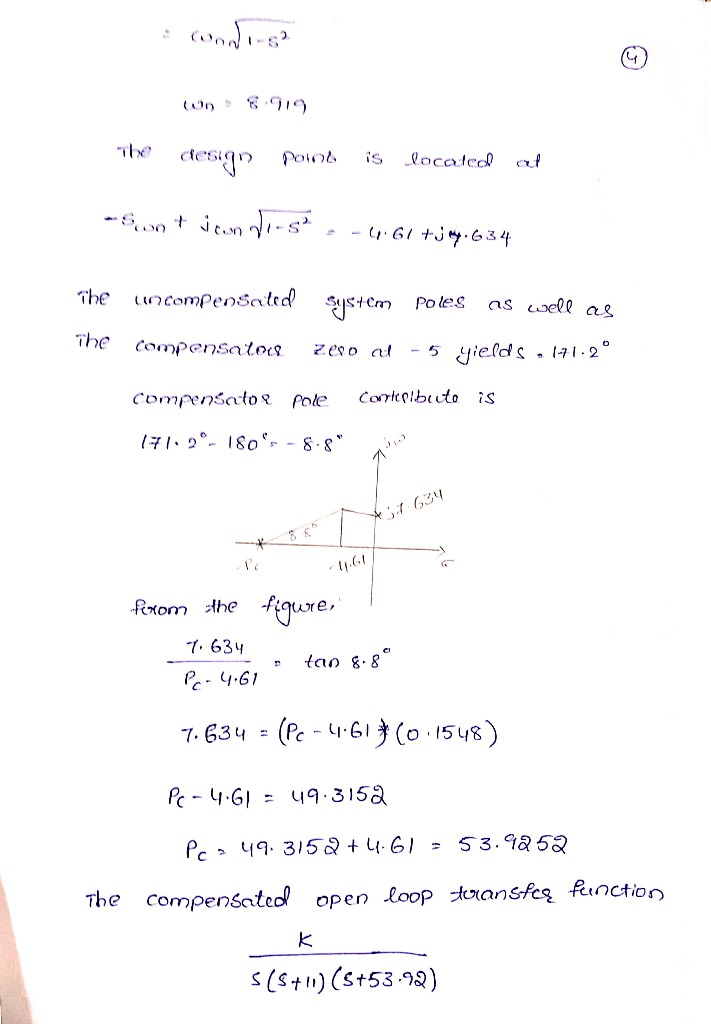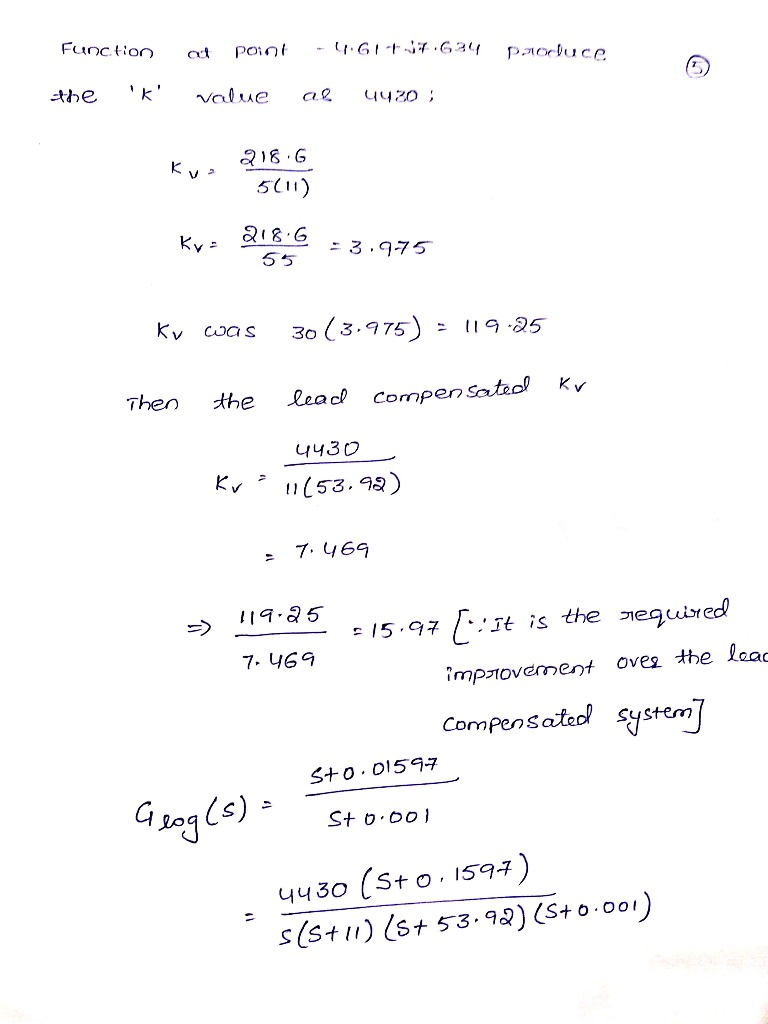#### Earn Coin

Coins can be redeemed for fabulous gifts.

Similar Homework Help Questions
• ### Problem (5): . The unity feedback system shown in Figure P9.1 with is operating with 10% overshoo...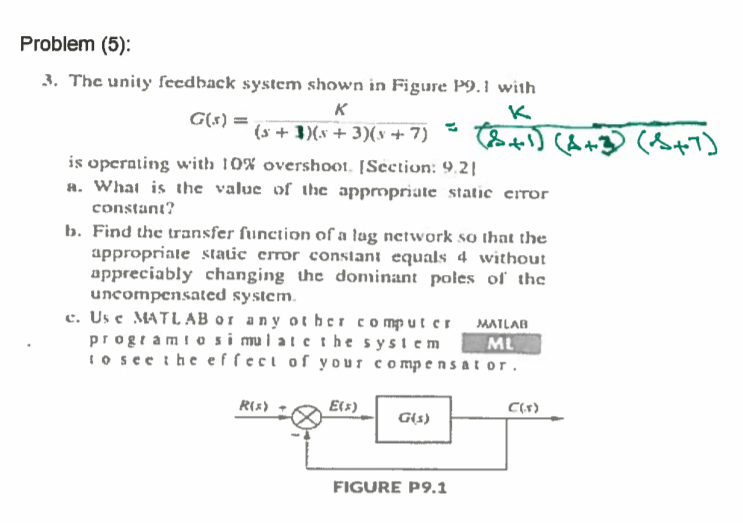Problem (5): . The unity feedback system shown in Figure P9.1 with is operating with 10% overshoot [Section: 9 21 R. Whai is the value of the appropriate static error constant? b. Find the transfer function of a lag network so that the appropriale static error constant equals 4 without appreciably changing he dominant poles of the uncompensated system e. Use MATLAB or any other computer MATLAD progtam to si mulatcthe system o seethe effect of your compensator ML C(F)...

• ### steps R(s) E(s) C(s) G(s) FIGURE P9.1 FIGURE P9.2 9. Consider the unity feedback system shown...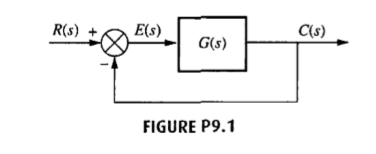steps R(s) E(s) C(s) G(s) FIGURE P9.1 FIGURE P9.2 9. Consider the unity feedback system shown in Figure P9.1 with [Section: 9.3] K G(s) (s+4)3 a. Find the location of the dominant poles to yield a 1.6 second settling time and an overshoot of 25%. b. If a compensator with a zero at -1 is used to achieve the conditions of Part a, what must the angular contribution of the compensator pole be? c. Find the location of the compensator...

• ### Please solve with detailed steps (NO MATLAB Solution).Thanks in advance 13. Consider the unity feedback system...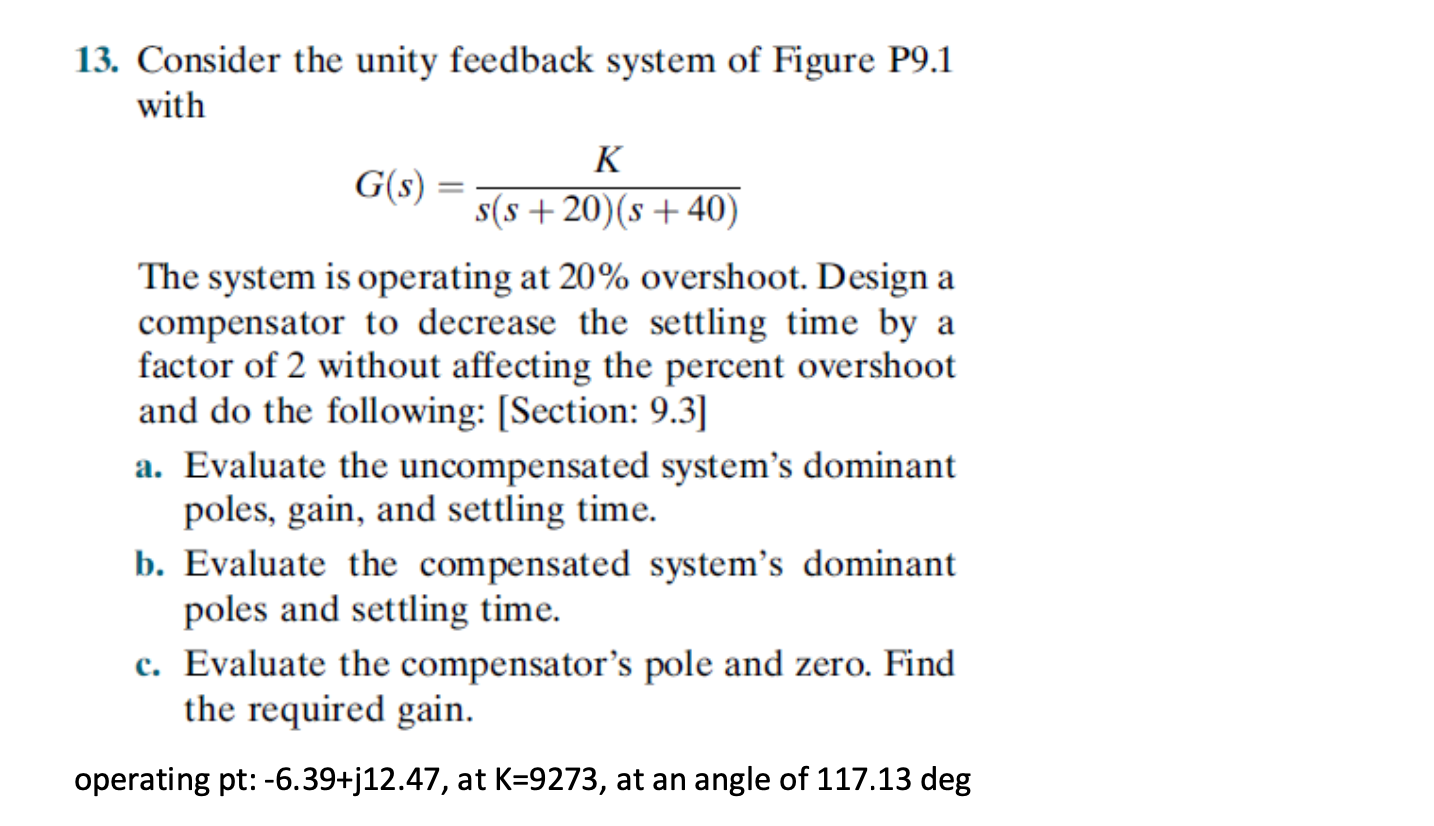Please solve with detailed steps (NO MATLAB Solution).Thanks in advance 13. Consider the unity feedback system of Figure P9.1 with K G(s) s(s +20)(s +40) The system is operating at 20% overshoot. Design a compensator to decrease the settling time by a factor of 2 without affecting the percent overshoot and do the following: (Section: 9.3] a. Evaluate the uncompensated system's dominant poles, gain, and settling time. b. Evaluate the compensated system's dominant poles and settling time. c. Evaluate the...

• ### 13. Consider the unity feedback system of Figure P11.1 with G(s) s(s+5s 20) The uncompensated sys...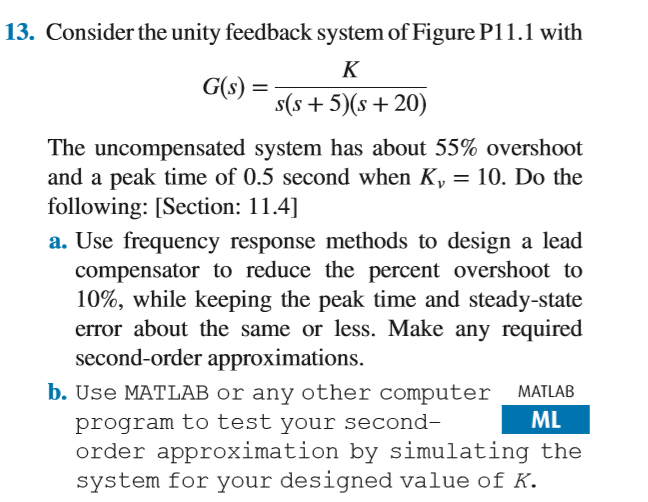13. Consider the unity feedback system of Figure P11.1 with G(s) s(s+5s 20) The uncompensated system has about 55% overshoot and a peak time of 0.5 second when K 10. Do the following: [Section: 11.4] . Use frequency response methods to design a lead compensator to reduce the percent overshoot to 10%, while keeping the peak time and steady-state error about the same or less. Make any required second-order approximations. b. Use MATLAB or any other computer MATLAB ML program...

• ### I8. Consider the unity feedback system of Figure P9.1 with G(s) s +3)(s +5) . Show...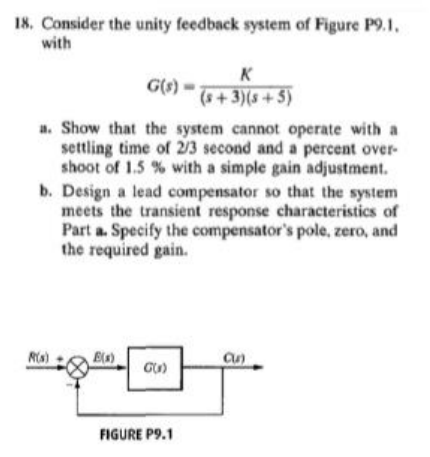I8. Consider the unity feedback system of Figure P9.1 with G(s) s +3)(s +5) . Show that the system cannot operate with a settling time of 2/3 second and a percent over- shoot of 1.5 % with a simple gain adjustment. b. Design a lead compensator so that the system meets the transient response characteristics of Part a.Specify the compensator's pole, zero, and the required gain. R) Cu) FIGURE P9.1

• ### Lag Compensator Design Using Root-Locus 2. Consider the unity feedback system in Figure 1 for G(s...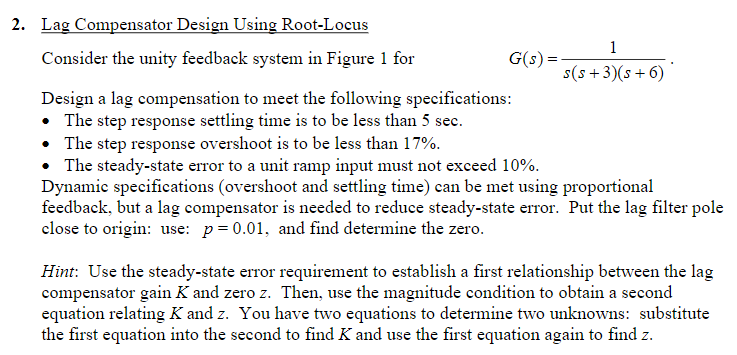Lag Compensator Design Using Root-Locus 2. Consider the unity feedback system in Figure 1 for G(s)- s(s+3(s6) Design a lag compensation to meet the following specifications The step response settling time is to be less than 5 sec. . The step response overshoot is to be less than 17% . The steady-state error to a unit ramp input must not exceed 10%. Dynamic specifications (overshoot and settling time) can be met using proportional feedback, but a lag compensator is needed...

• ### QUICK UPVOTE: As a control system engineer you have been asked to design a controller that...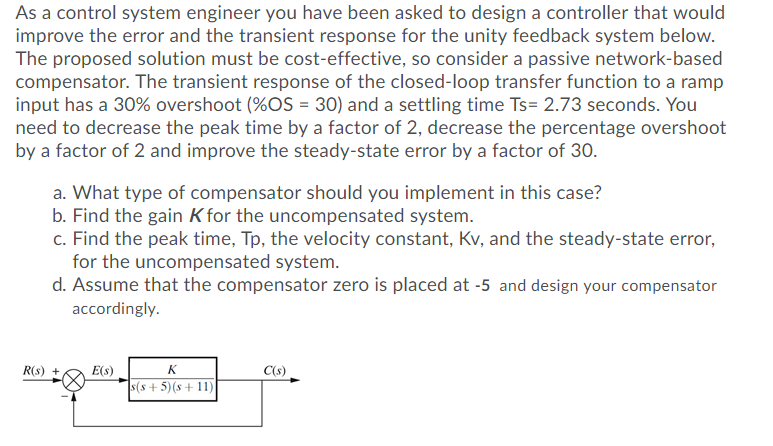QUICK UPVOTE: As a control system engineer you have been asked to design a controller that would improve the error and the transient response for the unity feedback system below. The proposed solution must be cost-effective, so consider a passive network-based compensator. The transient response of the closed-loop transfer function to a ramp input has a 30% overshoot (%OS = 30) and a settling time Ts= 2.73 seconds. You need to decrease the peak time by a factor of 2,...

• ### Question 1 (20 points) As a control system engineer you have been asked to design a...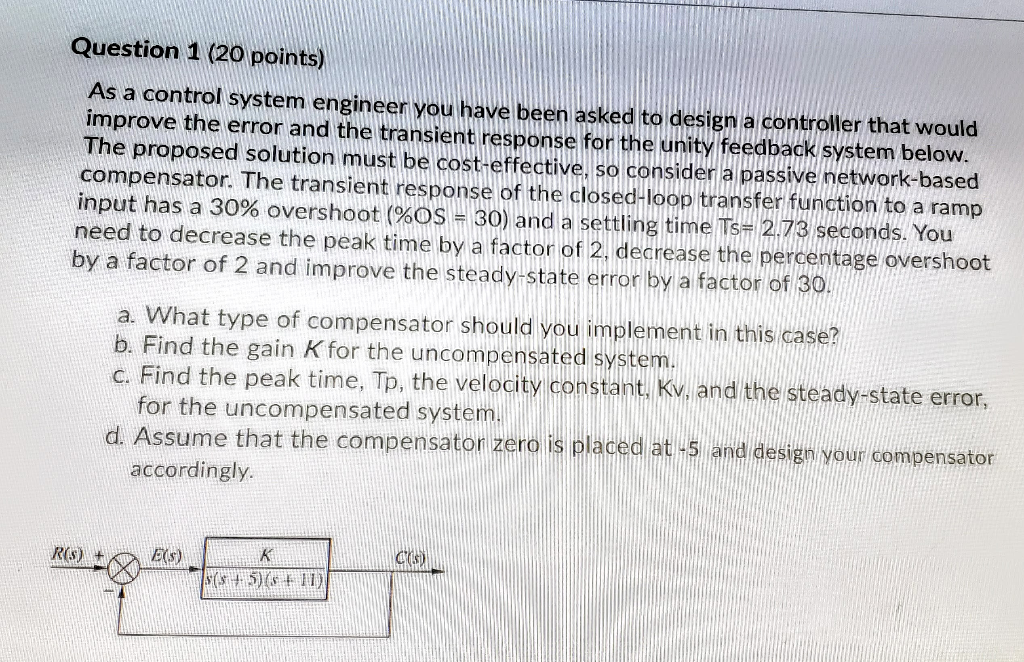Question 1 (20 points) As a control system engineer you have been asked to design a controller that would improve the error and the transient response for the unity feedback system below. The proposed solution must be cost-effective, so consider a passive network-based compensator. The transient response of the closed-loop transfer function to a ramp input has a 30% overshoot (%OS = 30) and a settling time Ts= 2.73 seconds. You need to decrease the peak time by a factor...

• ### PROBLEM: A unity feedback system with the forward transfer function K G(s) s(s+7) is operating with...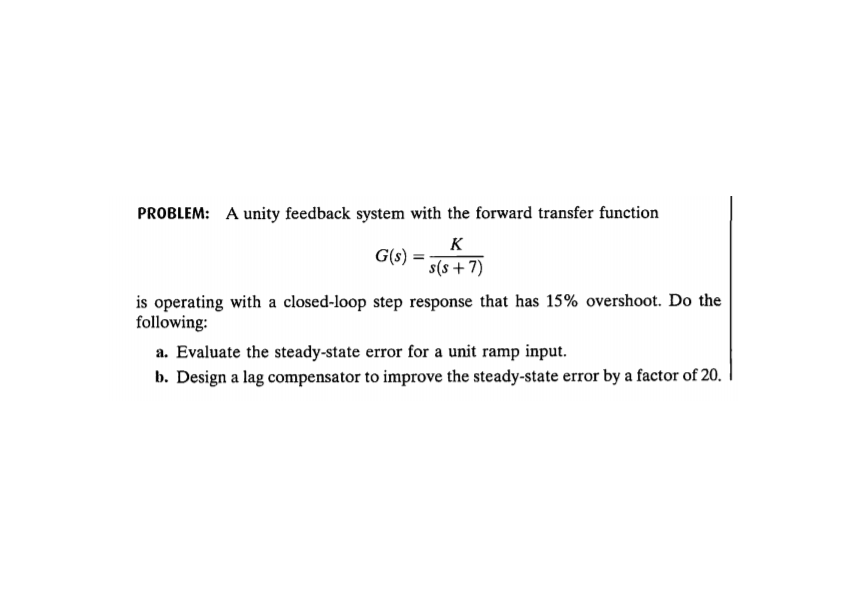PROBLEM: A unity feedback system with the forward transfer function K G(s) s(s+7) is operating with a closed-loop step response that has 15% overshoot. Do the following: a. Evaluate the steady-state error for a unit ramp input. b. Design a lag compensator to improve the steady-state error by a factor of 20. c. Evaluate the steady-state error for a unit ramp input to your compensated system. d. Evaluate how much improvement in steady-state error was realized.

• ### C(s) G(s) Figure 1: A block diagram for Problems 1-4 For the given unity feedback system with G(s...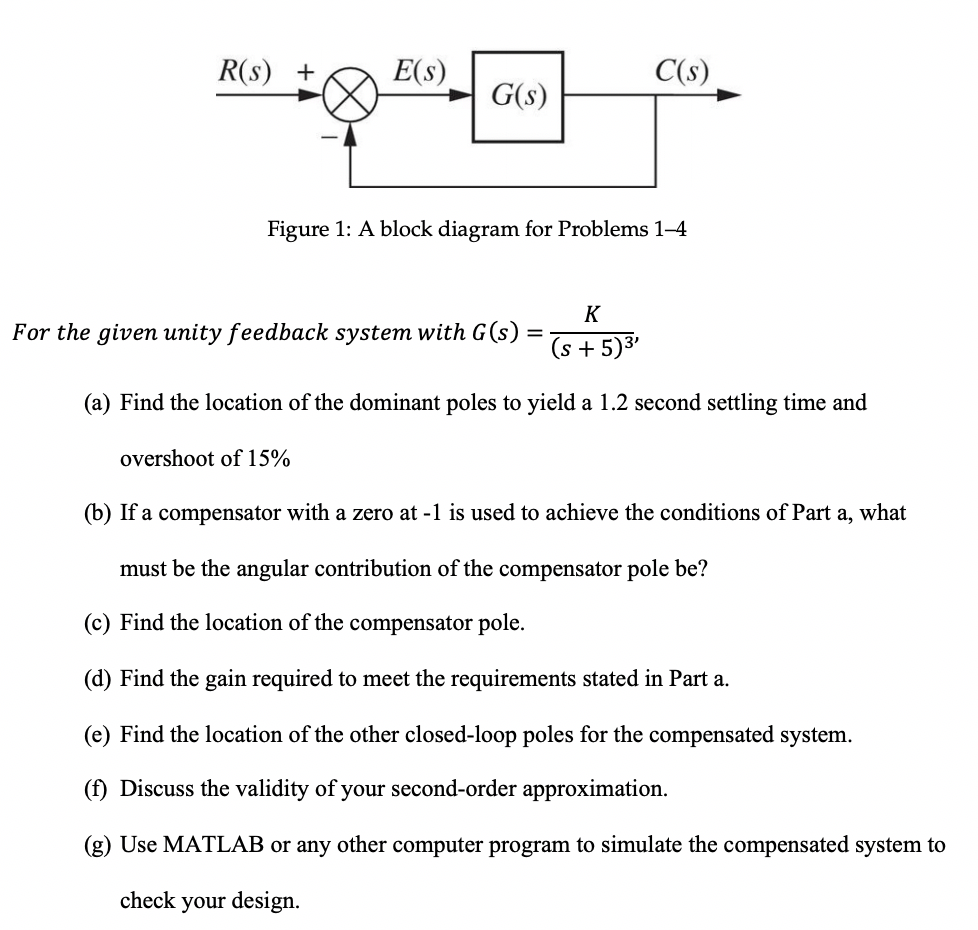C(s) G(s) Figure 1: A block diagram for Problems 1-4 For the given unity feedback system with G(s) - s 5)3' (a) Find the location of the dominant poles to yield a 1.2 second settling time and overshoot of 15% (b) If a compensator with a zero at-1 is used to achieve the conditions of Part a, what must be the angular contribution of the compensator pole be? (c) Find the location of the compensator pole. (d) Find the gain...

Free Homework App